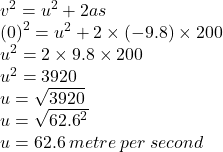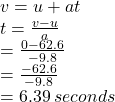Question

A body is thrown vertically upward and the height travelled by it is 200m , find the initial velocity and time required to reach maximum height ?

1.## Time(t)=6.39seconds

Solution,

Displacement(s)=200 m

Initial velocity (u)=?

Final velocity(v)=0 m/s^2

acceleration due to gravity(g)=9.8 m/s^2

now,Again,

Finding time taken,Hope this helps...